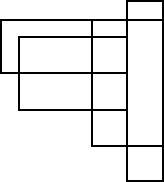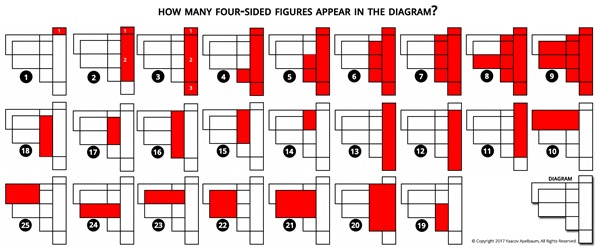# How many four-sided figures appear in the diagram?

There are a number of these geometric combinometrics problems around.  Here is a complete graphic solution to the one of the more common ones.

Question: How many four-sided figures appear in the diagram below?

1. 10
2. 16
3. 22
4. 25
5. 28## 2 thoughts on “How many four-sided figures appear in the diagram?”

1.answer is 22 not 25 as question was How many “four-sided” figures appear in the diagram below? and in shape above we more than “four-sided” shapes.

•Thanks for your comment Mahmoud, There are 25 unique 4 sided rectangle configurations in the diagram (marked in red).

Here is the summation method I used to arrive at this figure:
1’st row = 9
2’nd row = 9
3’rd row = 7
—————
Total = 25